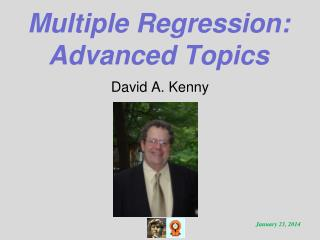DownloadDownload PresentationTélécharger la présentation- - - - - - - - - - - - - - - - - - - - - - - - - - - E N D - - - - - - - - - - - - - - - - - - - - - - - - - - -
##### Presentation Transcript

1. Multiple Regression:Advanced Topics David A. Kenny

2. Topics • You should already be familiar with Multiple Regression. • Rescaling • No intercept • Adjusted R2 • Bilinear Effects • Suppression

3. Rescaling a Predictor Imagine the following equation: Y = a + bX + E If Xʹ = d + eX, the new regression equation would be: Y = a – d(b/e) + (b/e)Xʹ + E The new intercept is a – d(b/e) and new slope for Xʹ is b/e. Note that if e = 1 which is what it equals with centering, then the new intercept is a – bd and the slope does not change.

4. What Changes? Coefficients intercept: almost always slope: only if the variable multiplied or divided Tests of coefficients intercept: almost always slope: no change R2 and predicted values no change

5. Rescaling the Criterion Imagine the following equation: Y = a + bX + E If Yʹ = d + eY, the new regression equation would be: Yʹ = ae + d + beX + E The new intercept is ae + d and new slope for Xʹ is be.

6. No Intercept • It is possible to run a multiple regression equation but fix the intercept to zero. • This is done for different reasons. • There may be a reason to believe that the intercept is zero: criterion a change score. • May want two intercepts, one for each level of a dichotomous predictor: two-intercept model.

7. Adjusted R2 The multiple correlation is biased, i.e. too large. We can adjust R2 for bias by [R2 – k/(N – 1)][(N – 1)/(N – k -1)] where N is the number of cases and k the number of predictors. If the result is negative, the adjusted R2 is set to zero. The adjustment is bigger if k is large relative to N. Normally, the adjustment is not made and the regular R2 is reported.

8. Bilinear or Piecewise Regression • Imagine you want the effect of X to change at a given value of X0. • Create two variables • X1 = X when X ≤ X0, zero otherwise • X2 = X when X > X0, zero otherwise • Regress Y on X1 and X2.

9. Y X0 X

10. Suppression It can occur that a predictor may have little or correlation with the criterion, but have a moderate to large regression coefficient.  For this to happen, two conditions must co-occur:  1) the predictor must be correlated relatively strongly with one (or more) other predictor and 2) that predictor must have a non-trivial coefficient. With suppression, because the suppressor is correlated with a predictor that has an effect on the criterion, the suppressor should correlate with the criterion.  But it is not correlated. To explain this, the suppressor has an effect that compensates for the lack of correlation.

11. Hypothetical Example Happiness and Sadness correlate -.75. Happiness correlates .4 with Work Motivation (WM) and Sadness correlates 0. The beta (standardized regression weight) for Happiness predicting WM is .914, and the beta for Sadness is .686. Sadness is the suppressor variable. It does not correlate with the criterion but it has a non-zero regression coefficient. Because Sadness correlates strongly negatively with Happiness and because Happiness correlates positively with WM, Sadness “should” correlate negatively with WM. Because it does not, it is given a positive regression coefficient.

12. Next Presentation • Example

13. Thank You!